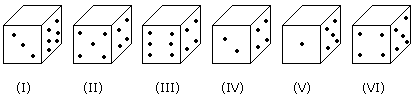# Verbal Reasoning - Dice - Discussion

### Discussion :: Dice - Dice 2 (Q.No.4)

Six dice with upper faces erased are as shows.The sum of the numbers of dots on the opposite face is 7.

4.

If the even numbers of dice have odd number of dots on their top faces and odd numbered dice have even of dots on their bottom faces, then what would be the total number of dots on their top faces?

 [A]. 12 [B]. 14 [C]. 16 [D]. 18

Explanation:

No. of dots on the top faces of the dice (II), (IV) and (VI) are 1, 1 and 1 respectively.

No. of dots on the top faces of the dice (I), (III) and (V) are 5, 5 and 3 respectively.

Required total = 5 + 5 + 3 + 1 + 1 + 1 = 16

 Ashok Kumar Nanda said: (Jul 27, 2013) How any body can be sure of the dots of top faces of II IV AND VI are 1, 1 and 1. I didn't understand, if any body can help me I will be very grateful.

 Anshu Goyal said: (May 9, 2014) Consider dice 1 to 6 individually. For (I), number opposite to 3 dots would be 4 dots (3+4=7), similarly number opposite to 6 dots would be 1 dot. Now, two faces are left ( Bottom and Top) and two numbers 2 and 5 are left (In a dice, there are 6 no.s 1,2,3,4,5,6). As given in the question, this (No. 1) is an odd numbered dice and the top face will contain odd number of dots (So 5 dots will be on the top). Similarly, number of dots on the top face of the remaining 5 dices will be evaluated and at the end, sum of all six numbers on top faces of 6 dices would be the answer.

 Mustaq said: (Aug 31, 2017) Does 12 and 3 have even numbers of that on their bomb on faces and the Das for 5th and 6th have a number of dots on the top faces then what will be the difference in the total number of top places between the two sets?

 Kundan said: (Aug 31, 2018) No of dots on the top faces of the dice (I) , (III) and (V) should be 1, 1 and 4 respectively as mentioned in the question.

 Shubham said: (Nov 24, 2018) The first statement itself says that opposite number form a sum of 7. So, 6+1=7, 4 + 3 = 7, 2 + 5= 7 are the opposite sides. So solving this, the answer is 14. Correct me if am wrong.

 Priyanka said: (Jun 8, 2020) The Correct answer is 16.